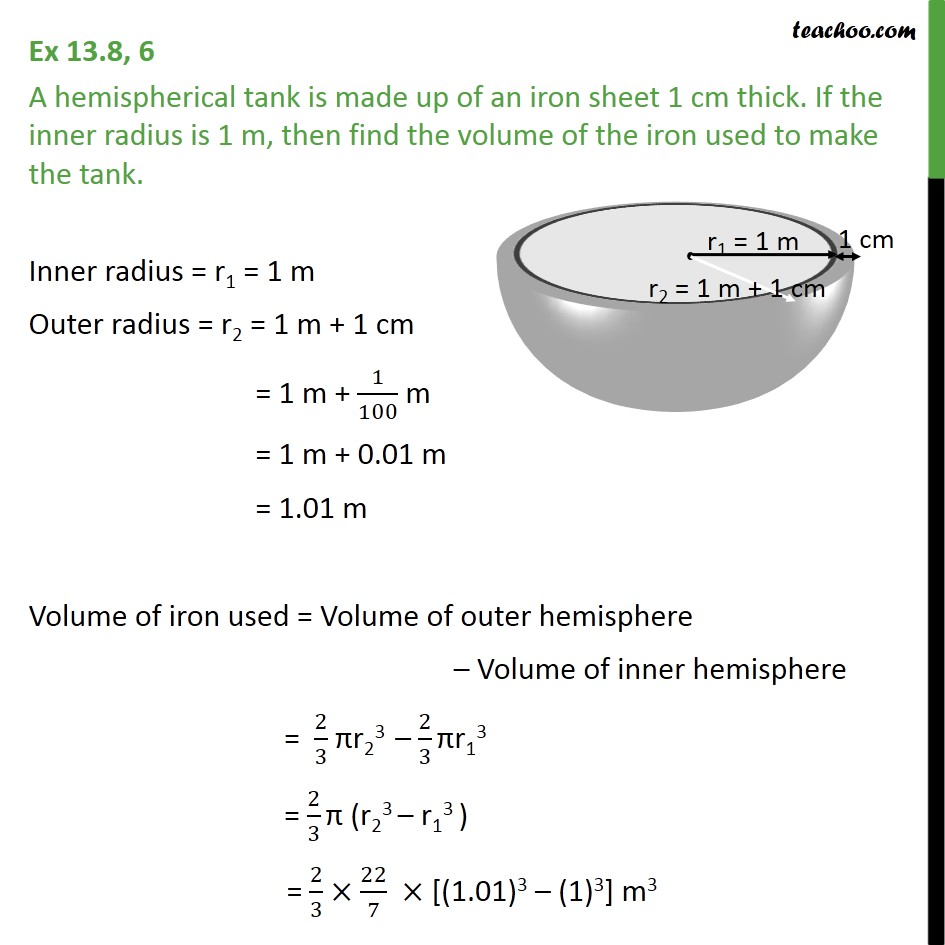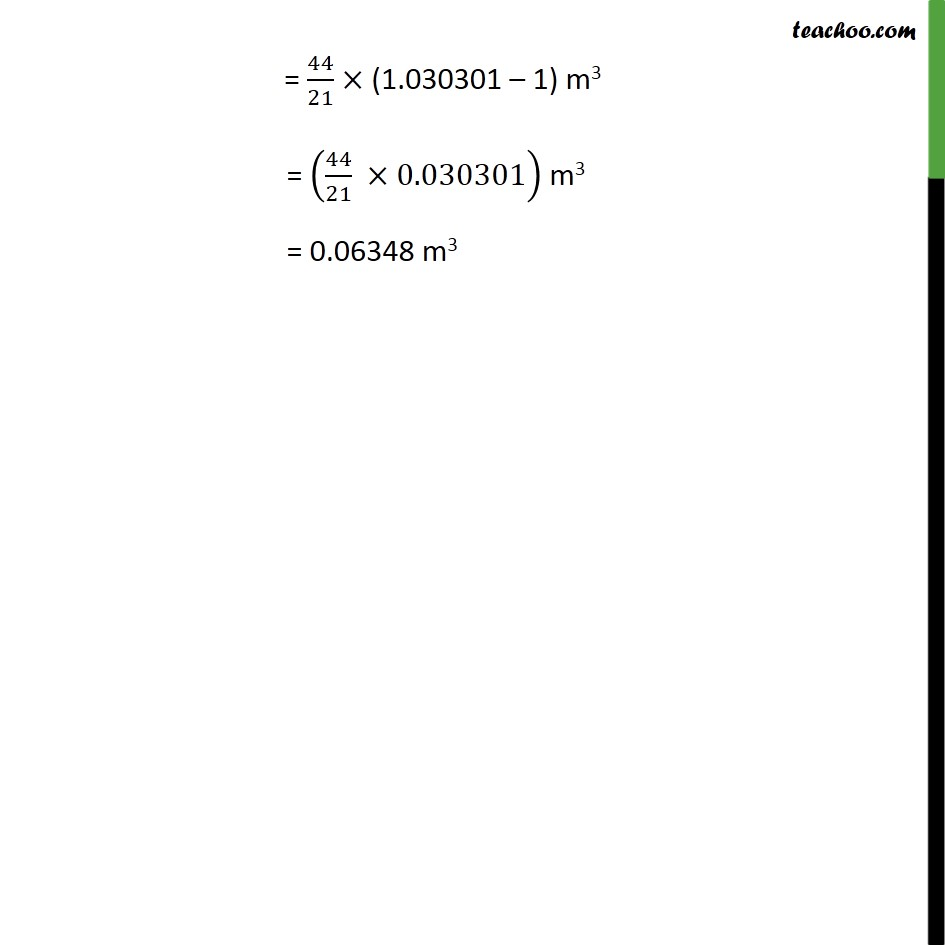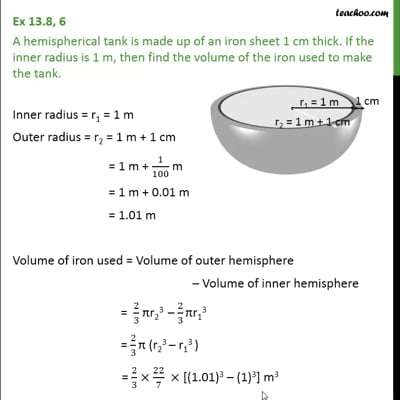Volume Of Hemisphere

Chapter 13 Class 9 Surface Areas and Volumes
Concept wiseThis video is only available for Teachoo black users

Introducing your new favourite teacher - Teachoo Black, at only ₹83 per month

### Transcript

Ex 13.8, 6 A hemispherical tank is made up of an iron sheet 1 cm thick. If the inner radius is 1 m, then find the volume of the iron used to make the tank. Inner radius = r1 = 1 m Outer radius = r2 = 1 m + 1 cm = 1 m + 1/100 m = 1 m + 0.01 m = 1.01 m Volume of iron used = Volume of outer hemisphere Volume of inner hemisphere = 2/3 r23 2/3 r13 = 2/3 (r23 r13 ) = 2/3 22/7 [(1.01)3 (1)3] m3 = 44/21 (1.030301 1) m3 = (44/21 0.030301) m3 = 0.06348 m3